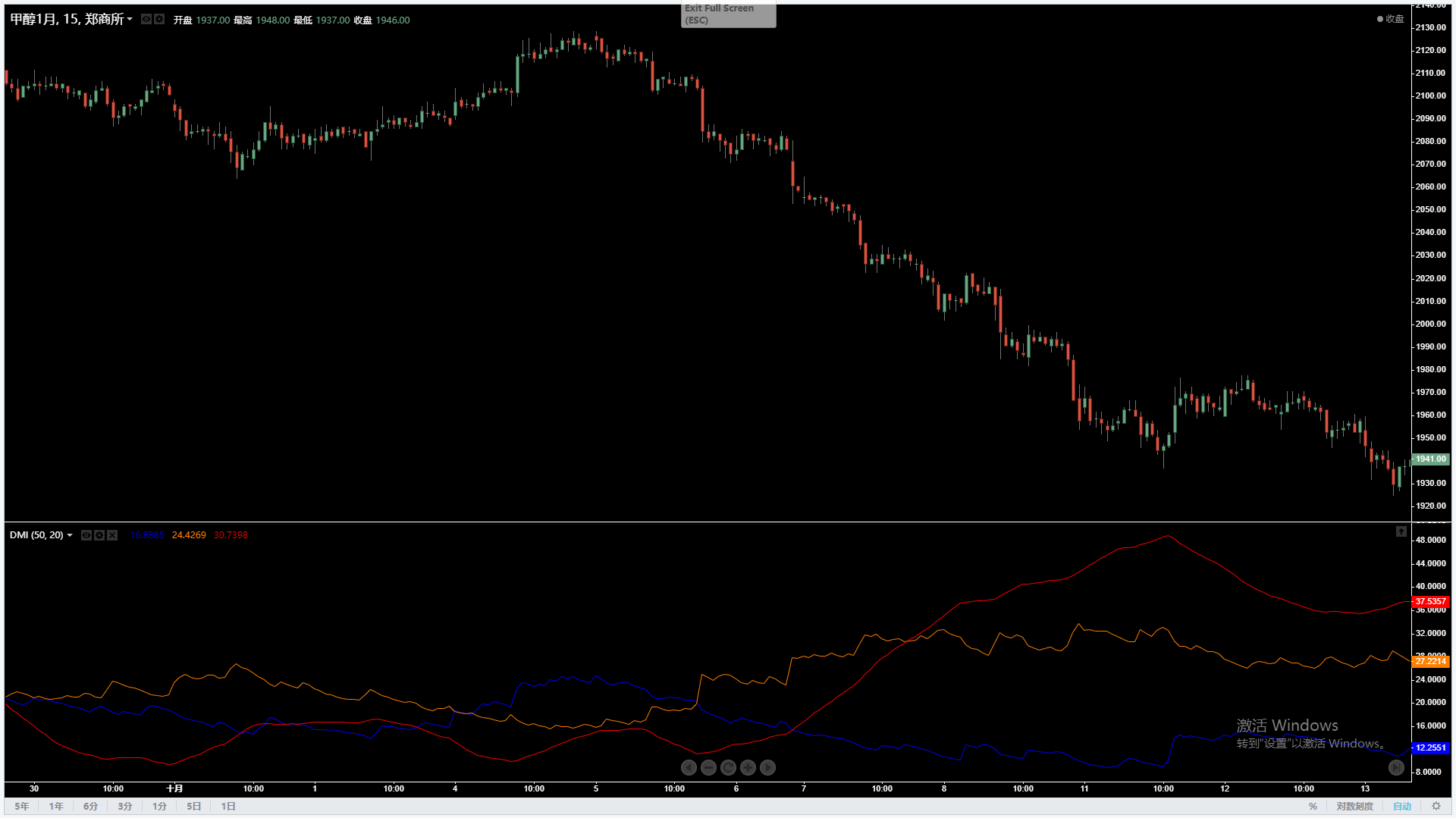# 利用平均趋向指数辅助MACD策略

1年前   阅读数 146 0• up：今天的最高价 – 昨天的最高价
• down：昨天的最低价 – 今天的最低价
• +DM：如果up大于max(down, 0)，则+DM等于up，否则等于零
• -DM：如果down大于max(up, 0)，则-DM等于down，否则等于零

• TR：max(今天最高价与今天最低价的差值，今天最高价与昨天收盘价差值的绝对值，今天最低价与昨天收盘价差值的绝对值)

• +DI(14)：+DM(14)/TR(14)*100
• -DI(14)：-DM(14)/TR(14)*100

• DX：((+DI14)- (-DI14)/(+DI14)+(-DI14))*100

• 多头开仓：DIF大于零轴
• 空头开仓：DIF小于零轴
• 多头平仓：DIF向下突破DEA
• 空头平仓：DIF向上突破DEA

``````# 回测配置
'''backtest
start: 2015-02-22 00:00:00
end: 2019-10-17 00:00:00
period: 1h
exchanges: [{"eid":"Futures_CTP","currency":"FUTURES"}]
'''

mp = 0  # 定义一个全局变量，用于控制虚拟持仓

# 判断数组是否上升
def is_up(arr):
arr_len = len(arr)
if arr[arr_len - 1] > arr[arr_len - 2] and arr[arr_len - 2] > arr[arr_len - 3]:
return True

# 判断数组是否下降
def is_down(arr):
arr_len = len(arr)
if arr[arr_len - 1] < arr[arr_len - 2] and arr[arr_len - 2] < arr[arr_len - 3]:
return True

# 判断两根两个数组是否金叉
def is_up_cross(arr1, arr2):
if arr1[len(arr1) - 2] < arr2[len(arr2) - 2] and arr1[len(arr1) - 1] > arr2[len(arr2) - 1]:
return True

# 判断两根两个数组是否死叉
def is_down_cross(arr1, arr2):
if arr1[len(arr1) - 2] > arr2[len(arr2) - 2] and arr1[len(arr1) - 1] < arr2[len(arr2) - 1]:
return True

# 程序主函数
def onTick():
exchange.SetContractType("rb000")  # 订阅期货品种
bar_arr = exchange.GetRecords()  # 获取K线数组
if len(bar_arr) < long + m + 1:  # 如果K线数组长度太小，就不能计算MACD，所以直接返回跳过
return
all_macd = TA.MACD(bar_arr, short, long, m)  # 计算MACD值，返回的是一个二维数组
dif = all_macd  # 获取DIF的值，返回一个数组
dif.pop()  # 删除DIF数组最后一个元素
dea = all_macd  # 获取DEA的值，返回一个数组
dea.pop()  # 删除DEA数组最后一个元素
last_close = bar_arr[len(bar_arr) - 1]['Close']  # 获取最新价格（卖价），用于开平仓
global mp  # 全局变量，用于控制虚拟持仓

# 开多单
if mp == 0 and dif[len(dif) - 1] > 0:
mp = 1  # 设置虚拟持仓的值，即有多单

# 开空单
if mp == 0 and dif[len(dif) - 1] < 0:
exchange.SetDirection("sell")  # 设置交易方向和类型
exchange.Sell(last_close - 1, 1)  # 开空单
mp = -1  # 设置虚拟持仓的值，即有空单

# 平多单
if mp == 1 and is_down_cross(dif, dea):
exchange.Sell(last_close - 1, 1)  # 平多单
mp = 0  # 设置虚拟持仓的值，即空仓

# 平空单
if mp == -1 and is_up_cross(dif, dea):
exchange.SetDirection("closesell")  # 设置交易方向和类型
mp = 0  # 设置虚拟持仓的值，即空仓

def main():
while True:
onTick()
Sleep(1000)
``````

``````import talib
import numpy as np
``````

``````# 把K线数组转换成最高价、最低价、收盘价数组，用于转换为numpy.array类型数据
def get_data(bars):
arr = [[], [], []]
for i in bars:
arr.append(i['High'])
arr.append(i['Low'])
arr.append(i['Close'])
return arr
``````

``````np_arr = np.array(get_data(bar_arr)) # 把列表转换为numpy.array类型数据，用于计算ADX的值
``````

``````adx1 = adx_arr[len(adx_arr) - 2]  # 倒数第二根K线的ADX值
``````

``````# 开多单
if mp == 0 and dif[len(dif) - 1] > 0 and adx1 > 40:
mp = 1  # 设置虚拟持仓的值，即有多单

# 开空单
if mp == 0 and dif[len(dif) - 1] < 0 and adx1 > 40:
exchange.SetDirection("sell")  # 设置交易方向和类型
exchange.Sell(last_close - 1, 1)  # 开空单
mp = -1  # 设置虚拟持仓的值，即有空单

# 平多单
exchange.Sell(last_close - 1, 1)  # 平多单
mp = 0  # 设置虚拟持仓的值，即空仓

# 平空单
exchange.SetDirection("closesell")  # 设置交易方向和类型
mp = 0  # 设置虚拟持仓的值，即空仓
``````

``````# 回测配置
'''backtest
start: 2015-02-22 00:00:00
end: 2019-10-17 00:00:00
period: 1h
exchanges: [{"eid":"Futures_CTP","currency":"FUTURES"}]
'''

# 导入库
import talib
import numpy as np

mp = 0  # 定义一个全局变量，用于控制虚拟持仓

# 把K线数组转换成最高价、最低价、收盘价数组，用于转换为numpy.array类型数据
def get_data(bars):
arr = [[], [], []]
for i in bars:
arr.append(i['High'])
arr.append(i['Low'])
arr.append(i['Close'])
return arr

# 判断两根两个数组是否金叉
def is_up_cross(arr1, arr2):
if arr1[len(arr1) - 2] < arr2[len(arr2) - 2] and arr1[len(arr1) - 1] > arr2[len(arr2) - 1]:
return True

# 判断两根两个数组是否死叉
def is_down_cross(arr1, arr2):
if arr1[len(arr1) - 2] > arr2[len(arr2) - 2] and arr1[len(arr1) - 1] < arr2[len(arr2) - 1]:
return True

# 程序主函数
def onTick():
exchange.SetContractType("rb000")  # 订阅期货品种
bar_arr = exchange.GetRecords()  # 获取K线数组
if len(bar_arr) < long + m + 1:  # 如果K线数组长度太小，就不能计算MACD，所以直接返回跳过
return
all_macd = TA.MACD(bar_arr, short, long, m)  # 计算MACD值，返回的是一个二维数组
dif = all_macd  # 获取DIF的值，返回一个数组
dif.pop()  # 删除DIF数组最后一个元素
dea = all_macd  # 获取DEA的值，返回一个数组
dea.pop()  # 删除DEA数组最后一个元素

last_close = bar_arr[len(bar_arr) - 1]['Close']  # 获取最新价格（卖价），用于开平仓
global mp  # 全局变量，用于控制虚拟持仓

# 开多单
if mp == 0 and dif[len(dif) - 1] > 0 and adx1 > 40:
mp = 1  # 设置虚拟持仓的值，即有多单

# 开空单
if mp == 0 and dif[len(dif) - 1] < 0 and adx1 > 40:
exchange.SetDirection("sell")  # 设置交易方向和类型
exchange.Sell(last_close - 1, 1)  # 开空单
mp = -1  # 设置虚拟持仓的值，即有空单

# 平多单
exchange.Sell(last_close - 1, 1)  # 平多单
mp = 0  # 设置虚拟持仓的值，即空仓

# 平空单
exchange.SetDirection("closesell")  # 设置交易方向和类型
mp = 0  # 设置虚拟持仓的值，即空仓

def main():
while True:
onTick()
Sleep(1000)

``````

我有话说: